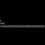# What is the buoyant and viscous force acting on a ball in free fall?

was thinking about a situation were I have a long cylindrical jar with some viscous liquid in it. I also have a spherical ball with me. I drop the ball into the liquid inside the jar with some initial velocity. Just after that(before the ball acquires terminal velocity) I drop the system(the jar with the liquid and ball) from certain height.

(A) What would be the buoyant and viscous force in free fall compared to those that were acting before free fall?

(B) Will the answer change if I drop the ball(with some initial velocity) into the jar and drop the jar simultaneously?

(C)I somehow got the courage to jump along with the jar(without the ball in it). Now, I put the ball inside the cylinder with some initial velocity.Will the answer change now? (Remember that the whole system along with me is in free fall).

I tried to answer my own question like this: In all these cases I think the buoyant force will be zero. By Archimedes Principle it is equal to weight of the liquid displaced.But, as something in free fall has zero weight there will be no weight for the liquid displaced and hence no buoyant force on the ball.

From Stokes law we know that the viscous force is directly proportional to the velocity of the ball in the liquid. Consider a frame of reference R to be moving at an acceleration g downwards. Thus, in all these situations the acceleration of the ball and the cylinder due to free fall is zero relative to R. Hence, from R's perspective the viscous force would the same to that when the system was at rest.

I'm able to explain about the viscous force only in this way. What will be the viscous force for a man standing on the ground. Please help me with your ideas about the situation.Note by Rajath Krishna R
6 years, 8 months ago

This discussion board is a place to discuss our Daily Challenges and the math and science related to those challenges. Explanations are more than just a solution — they should explain the steps and thinking strategies that you used to obtain the solution. Comments should further the discussion of math and science.

When posting on Brilliant:

• Use the emojis to react to an explanation, whether you're congratulating a job well done , or just really confused .
• Ask specific questions about the challenge or the steps in somebody's explanation. Well-posed questions can add a lot to the discussion, but posting "I don't understand!" doesn't help anyone.
• Try to contribute something new to the discussion, whether it is an extension, generalization or other idea related to the challenge.

MarkdownAppears as
*italics* or _italics_ italics
**bold** or __bold__ bold
- bulleted- list
• bulleted
• list
1. numbered2. list
1. numbered
2. list
Note: you must add a full line of space before and after lists for them to show up correctly
paragraph 1paragraph 2

paragraph 1

paragraph 2

[example link](https://brilliant.org)example link
> This is a quote
This is a quote
    # I indented these lines
# 4 spaces, and now they show
# up as a code block.

print "hello world"
# I indented these lines
# 4 spaces, and now they show
# up as a code block.

print "hello world"
MathAppears as
Remember to wrap math in $$ ... $$ or $ ... $ to ensure proper formatting.
2 \times 3 $2 \times 3$
2^{34} $2^{34}$
a_{i-1} $a_{i-1}$
\frac{2}{3} $\frac{2}{3}$
\sqrt{2} $\sqrt{2}$
\sum_{i=1}^3 $\sum_{i=1}^3$
\sin \theta $\sin \theta$
\boxed{123} $\boxed{123}$